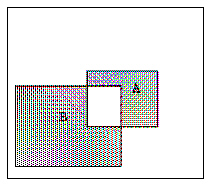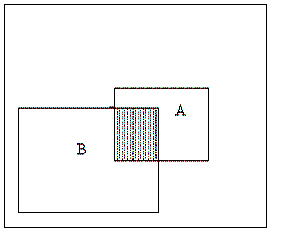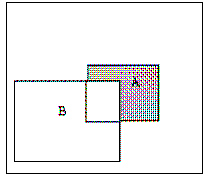Name:    Chapter 6 - Probability

Multiple Choice
Identify the choice that best completes the statement or answers the question.

1.

An assignment of probability must obey which of the following?
 a. The probability of any event must be a number between 0 and 1, inclusive. b. The sum of all the probabilities of all outcomes in the sample space must be exactly 1. c. The probability of an event is the sum of the outcomes in the sample space which make up the event. d. All of the above. e. A and B only.

2.

Students at University X must be in one of the class ranks—freshman, sophomore, junior, or senior. At University X, 35% of the students are freshmen and 30% are sophomores. If a student is selected at random, the probability that her or she is either a junior or a senior is
 a. 30%. b. 35%. c. 65%. d. 70%.

In a particular game, a fair die is tossed. If the number of spots showing is either four or five, you win \$1. If the number of spots showing is six, you win \$4. And if the number of spots showing is one, two, or three, you win nothing. You are going to play the game twice.

3.

The probability that you win \$4 both times is
 a. 1/6 b. 1/3 c. 1/36 d. 1/4 e. 1/12

4.

The probability that you win money at least once in the two games is
 a. 0.75 b. 0.5 c. 0.25 d. 0.125 e. 0.1

An event A will occur with probability 0.5. An event B will occur with probability 0.6. The probability that both A and B will occur is 0.1.

5.

The conditional probability of A given B is
 a. 0.5. b. 0.3. c. 0.2. d. 1/6. e. cannot be determined from the information given.

6.

Experience has shown that a certain lie detector will show a positive reading (indicates a lie) 10% of the time when a person is telling the truth and 95% of the time when a person is lying. Suppose that a random sample of 5 suspects is subjected to a lie detector test regarding a recent one-person crime. Then the probability of observing no positive reading if all suspects plead innocent and are telling the truth is
 a. 0.409 b. 0.735 c. 1e-05 d. 0.59 e. 0.99999

7.

If you buy one ticket in the Provincial Lottery, then the probability that you will win a prize is 0.11. If you buy one ticket each month for five months, what is the probability that you will win at least one prize?
 a. 0.55 b. 0.5 c. 0.44 d. 0.45 e. 0.56

8.

Suppose that A and B are two independent events with P(A) = .2 and P(B) = .4.
P(A Ç Bc) is
 a. 0.08. b. 0.12. c. 0.52. d. 0.60.

9.

A plumbing contractor puts in bids in on two large jobs. Let the event that the contractor wins the first contract be A and the event that the contractor wins the second contract be B. Which of the Venn diagrams has shaded the event that the contractor wins exactly one of the contracts?
 a.c.b.d.10.

A die is loaded so that the number 6 comes up three times as often as any other number.  What, then, is the probability of rolling a 6?
 a. .125 b. .250 c. .375 d. .500 e. None of the above.